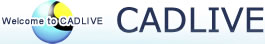### Genetic modification of flux for flux prediction of mutants

Bioinformatics, 2009

### Instruction manuals of Genetic Modification of Flux

The Matlab programs for the modified Control Effective flux (mCEF), Quadratic Programming (QP) for the optimization of Elementary Mode Coefficients (EMCs) and Enzyme Control Flux (ECF) are presented. The version of Matlab is 7.4.0.287 (R2007a).

#### 1. Modified Control Effective Flux (mCEF)

mcef1.m is the main program for mCEF in Matlab. The parameters necessary for mCEF are shown below: em: elementary mode matrix; (reactionsxelementary modes) ni: the row number of the substrate uptake reaction in EM matrix; cellfunc: a row vector includes the serial numbers of the reactions for cellular functions in EM matrix, such as biomass formation or ATP drain. eta: a column vector for the relative enzyme activity of a mutant to wild type for the genetically modified genes. All of the parameters are saved in an input data file. For example, the input data file is mcef1.mat, where all the parameters are available in the right format.
Run the program in Matlab,
>> mcef1(em,ni,cellfunc,eta)

The output variable of rge shows the relative ratios of the gene expressions in a mutant to those in wild type. rge is saved in mcefrge.mat file.

#### 2. Quadratic programming for the optimization of Elementary Mode Coefficients (EMCs)

A quadratic programming (QP) is applied to the optimization of EMCs. Qpexample.mat is the input data file for the QP optimization. a is a submatrix of the EM matrix (reactionsxelementary modes) b is a row vector of the determined fluxes (1xdetermined fluxes).

Run the program in Matlab:
>> qpexample(a,b)

The output variable of emcs shows the optimal elementary mode coefficients for wild type. It is saved in qpemcs.mat.

#### 3. Enzyme Control Flux (ECF)

ecf1.m is the program for the metabolic flux prediction for genetic modified mutants in Genetic Modified Flux (GMF). function ecf1(em,emcs,ni,rge, sflux)
em: elementary mode matrix; (reactions x elementary modes)
emcs: elementary mode coefficients (elementary modes x 1): saved in qpemcs.mat
ni: the row number of the substrate uptake reaction in EM matrix
sflux: flux of substrate uptake
rge: mCEF ratios calculated by mCEF. (1 x reactions): saved in mcefrge.mat

There are three input data files which are ecf1.mat, mcefrge.mat and qpemcs.mat for different parameters for calculation. em, ni and sflux are saved in ecf1.mat. rge is saved in mcefrge.mat which is obtained by mcef1.m. emcs is saved in qpemcs.mat which is obtained by qpexample.m All of the parameters are all available in the right format.

The output variable of fluxcalu shows the flux distribution of a mutant calculated by ECF. It is saved in ecfflux.mat
In Matlab,